# Change problem

The change-making problem, often simply known as change, occurs in two distinct but related flavours. Given a set of natural numbers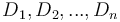$D_1, D_2, ..., D_n$ (the denominations) and a natural number$T$ (the target amount for which to make change):

• Optimization problem: Make change for the target amount using as few total coins as possible. Formally, find nonnegative integers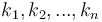$k_1, k_2, ..., k_n$ that minimize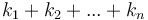$k_1 + k_2 + ... + k_n$ subject to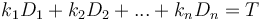$k_1 D_1 + k_2 D_2 + ... + k_n D_n = T$, or determine that this is impossible.
• Counting problem: Find the total number of different ways to make change for the target amount. Formally, count the number of tuples of nonnegative integers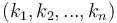$(k_1, k_2, ..., k_n)$ that satisfy$k_1 D_1 + k_2 D_2 + ... + k_n D_n = T$.

The former, the optimization problem, should be very familiar. It is a problem that cashiers solve when (for example) you hand them a five-dollar bill for a purchase of \$3.44, and they have to find a way to give you back \$1.56 in change, preferably with as few coins as possible. It is a well-studied problem that is extensively treated in the literature. The counting problem is somewhat more exotic; it arises mostly as a mathematical curiosity, often in the form of the puzzle: "How many ways are there to make change for a dollar?" It is also not often encountered in the literature, but it is discussed here because it occasionally appears in algorithmic programming competitions.

## Discussion of complexity

The corresponding decision problem, which simply asks us to determine whether or not making change is possible with the given denominations (which it might not be, if we are missing the denomination 1) is known to be NP-complete. It follows that the optimization and counting problems are both NP-hard (e.g., because the result of 0 for the counting problem answers the decision problem in the negative, and any nonzero value answers it in the affirmative).

However, as we shall see, a simple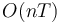$O(nT)$ solution exists for both versions of the problem. Why then are these problems not in P? The answer is that the size of the input required to represent the number$T$ is actually the length of the number$T$, which is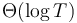$\Theta(\log T)$ when$T$ is expressed in binary (or decimal, or whatever). Thus, the time and space required by the algorithm is actually$O(n 2^{\lg T})$, that is, exponential in the size of the input. (This simplified analysis does not take into account the sizes of the denominations, but captures the essence of the argument.) This algorithm is then said to be pseudo-polynomial. No true polynomial-time algorithm is known (and, indeed, none will be found unless it turns out that P = NP).

## Greedy algorithm

Many real-world currency systems admit a greedy solution to the optimization version of the change problem. This algorithm is as follows: repeatedly choose the largest denomination that is less than or equal to the target amount, and use it, that is, subtract it from the target amount, and then repeat this procedure on the reduced value, until the target amount decreases to zero. For example, with Canadian currency, we can greedily make change for \$0.63 as follows: the largest denomination that fits into \$0.63 is \$0.25, so we subtract that (and thus resolve to use a \$0.25 coin); we are left with \$0.38, and take out another \$0.25, so we subtract that again to obtain \$0.13 (so that we have used two \$0.25 coins so far); now the largest denomination that fits is \$0.10, so we subtract that out, leaving us with \$0.03; and then we subtract three \$0.01 coins, leaving us with \$0.00, at which point the algorithm terminates; so we have used six coins (two \$0.25 coins, one \$0.10 coin, and three \$0.01 coins).

It turns out that the greedy algorithm always gives the correct result for both Canadian and United States currencies (the proof is left as an exercise for the reader). There are various other real-world currency systems for which this is also true. However, there are simple examples of sets of denominations for which the greedy algorithm does not give a correct solution. For example, with the set of denominations${1, 3, 4}$, the greedy algorithm will change 6 as 4+1+1, using three coins, whereas the correct minimal solution is obviously 3+3. There are also cases in which the greedy algorithm will fail to make change at all (consider what happens if we try to change 6 using the denominations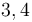${3, 4}$). This usually does not occur in real-world systems because they tend to have denominations that are quite a bit more "spaced out".

Obviously, there is no greedy solution to the counting problem.

## Dynamic programming solution

The optimization problem exhibits optimal substructure, in the sense that if we remove any coin of value$D_i$ from the optimal means of changing$T$, then the set of coins remaining is an optimal means of changing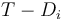$T - D_i$. This is because if this were not so; that is, there existed a means of changing$T - D_i$ that used fewer coins than what we obtained by removing the coin$D_i$ from our supposed optimal change for$T$, then we could just add the coin$D_i$ back in and get change for the original amount$T$ in fewer coins, a contradiction. Therefore, if we let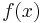$f(x)$ denote the minimal number of coins required to change amount$x$, then we can write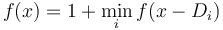$f(x) = 1 + \min_i f(x - D_i)$; we consider all possible minimal solutions to$x$ minus one coin, and take the best one and add that coin back in to get minimal change for$x$. The base case is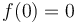$f(0) = 0$; obviously, 0 coins are required to make change for 0. See the DP article for details and an implementation.

The counting problem is more subtle. We cannot approach it in quite the same way as we approach the optimization problem, because the counting problem does not exhibit disjoint substructure when it is "sliced" this way. For example, if the denominations are 2 and 3, and the target amount is 5, then we might try to conclude that the number of ways of changing 5 is the number of ways of changing 2 plus the number of ways of changing 3, because we can either add a coin of value 2 to any way of changing 3 or add a coin of value 3 to any way of changing 2. Alas, this gives the incorrect answer that there are 2 ways of changing 5, whereas in actual fact we double-counted the solution 2+3 and there is, in fact, only one way to change 5.

The solution in this case is to compute the function$f(x, n)$, the number of ways to make change for$x$ using only the first$n$ denominations (and not necessarily all of them). The base case is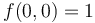$f(0, 0) = 1$ and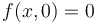$f(x, 0) = 0$ for all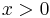$x > 0$; we don't need any coins to make change for the amount 0, and there is exactly one way to make change for 0, that is, the zero tuple. On the other hand, we obviously cannot change any nonzero amount if we are not allowed to use any denominations at all.

Now here comes the disjoint and exhaustive substructure. To make change for$x$ using only the first$n$ denominations, we have two disjoint and exhaustive options: either we can use at least one coin of denomination$D_n$, or we can use none at all. The number of ways of making change for$x$ using no coins of denomination$D_n$ is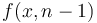$f(x, n-1)$. As for the ways of using at least one coin of denomination$D_n$, they can be put in one-to-one correspondence with ways of making change for$x-D_n$ with only the first$n$ coins (simply by addition or removal of one coin of denomination$D_n$). We conclude that$f(x, n) = f(x, n-1) + f(x - D_n, n)$.

We can implement this algorithm in$O(T)$ space by running it one column at a time; that is, the value$f(x, n)$ does not depend on any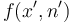$f(x', n')$ with$n' < n - 1$, so we only need to keep the last two columns at any given point. In fact, we do not even need two columns; the only value from the previous column that the computation of$f(x, n)$ requires is$f(x, n-1)$, so we can overwrite in-place with the new value (the old value will never be needed for the rest of this column). Here is pseudocode:

```input T, n, array D
dp ← 1
for i ∈ [1..T]
dp[i] ← 0
for j ∈ [1..n]
for i ∈ [D[j]..T]
dp[i] ← dp[i] + dp[i-D[j]]
print dp[T]
```

Should we actually wish to print out all ways of making change, the corresponding recursive descent algorithm would be a sound choice.[elaborate?]

## Notes and References

1. The Canadians have a \$2.00 coin, a \$1.00 coin, a \$0.25 coin, a \$0.10 coin, a \$0.05 coin, and a \$0.01 coin. The solution to this problem in Canadian currency is one \$1.00 coin, two \$0.25 coins, one \$0.05 coin, and one \$0.01 coin.
2. The denominations in circulation in the United States are the same as those in Canada, except for the absence of a \$2.00 coin and the presence of a \$0.50 coin. In United States currency, the answer is 293 if the trivial solution consisting of a single one-dollar coin is counted, or 292 if it is not.
3. G. S. Lueker. (1975). Two NP-complete problems in nonnegative integer programming. Technical Report 178, Computer Science Laboratory, Princeton University. (The authors were not able to obtain a copy of this paper, but in the literature it is invariably cited to back up the claim that change is NP-complete.)